StatLect

# Factorization of joint probability density functions

This lecture discusses how to factorize the joint probability density function of two continuous random variables (or random vectors)andinto two factors:

1. the conditional probability density function ofgiven;

2. the marginal probability density function of.## The factorization

The factorization, which has already been discussed in the lecture entitled Conditional probability distributions, is formally stated in the following proposition.

Proposition (factorization) Letbe a continuous random vector with supportand joint probability density function. Denote bythe conditional probability density function ofgivenand bythe marginal probability density function of. Then,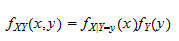for anyand.

## A factorization method

When we know the joint probability density functionand we need to factorize it into the conditional probability density functionand the marginal probability density function, we usually proceed in two steps:

1. marginalizeby integrating it with respect toand obtain the marginal probability density function;

2. dividebyand obtain the conditional probability density function(of course this step makes sense only when).

In some cases, the first step (marginalization) can be difficult to perform. In these cases, it is possible to avoid the marginalization step, by making a guess about the factorization ofand verifying whether the guess is correct with the help of the following proposition.

Proposition (factorization method) Suppose there are two functionsandsuch that

1. for anyand, the following holds: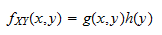2. for any fixed,, considered as a function of, is a probability density function

Then,Proof

The proof covers the case in whichandare random variables. The proof for the case in which they are random vectors is a straightforward generalization of this proof. The marginal probability density ofsatisfies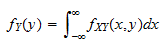therefore, by property 1 above:where the last equality follows from the fact that, for any fixed,, considered as a function of, is a probability density function and the integral of a probability density function overequals. Therefore,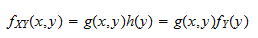which, in turn, implies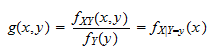Thus, whenever we are given a formula for the joint density functionand we want to find the marginal and the conditional functions, we have to manipulate the formula and express it as the product of:

1. a function ofandthat is a probability density function infor all values of;

2. a function ofthat does not depend on.

Example Let the joint density function ofandbeThe joint density can be factorized as follows:where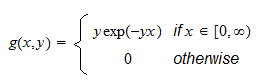andNote thatis a probability density function infor any fixed(it is the probability density function of an exponential random variable with parameter). Therefore,